# Related Videos

## Solve the logarithmic equation $\log \left(4x^2\right)=128$

Go!
1
2
3
4
5
6
7
8
9
0
a
b
c
d
f
g
m
n
u
v
w
x
y
z
.
(◻)
+
-
×
◻/◻
/
÷
2

e
π
ln
log
log
lim
d/dx
Dx
|◻|
=
>
<
>=
<=
sin
cos
tan
cot
sec
csc

asin
acos
atan
acot
asec
acsc

sinh
cosh
tanh
coth
sech
csch

asinh
acosh
atanh
acoth
asech
acsch

### Videos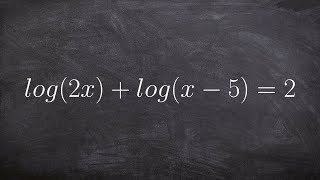### Tutorial - Solving logarithmic equations ex 10, log(2x)+log(x-5)=2

https://www.youtube.com/watch?v=fzjoQXBd0uU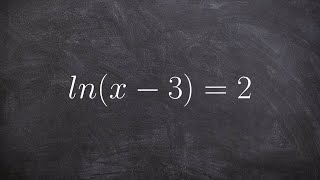### Calculus - Solving an natural logarithmic equation using properties of logs, ln(x - 3) = 2

https://www.youtube.com/watch?v=13GIbhWT7W0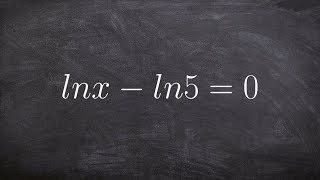### Pre-Calculus - How to solve a logarithmic equation by using the properties of logs lnx - ln5 = 0

https://www.youtube.com/watch?v=06jAbsXGXP4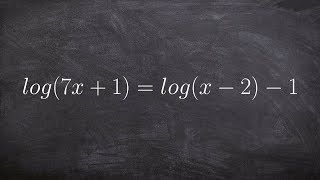### Tutorial - Solving logarithmic equations ex 14, log(7x+1)=log(x-2)-1

https://www.youtube.com/watch?v=v7Zsmb17CUc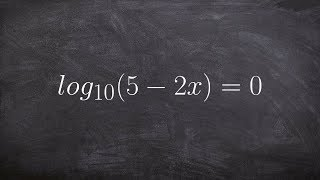### Tutorial - Solving logarithmic equations ex 5, log(5-2x)=0

https://www.youtube.com/watch?v=NaxzQH3eqcw### Solving Logarithmic Equations With Logs on Both Sides, Ln, e, Square Roots - Algebra

https://www.youtube.com/watch?v=G_kHwdrxyeU
$\log\left(4X^2\right)=128$

### Main topic:

Logarithmic Equations

~ 0.04 s